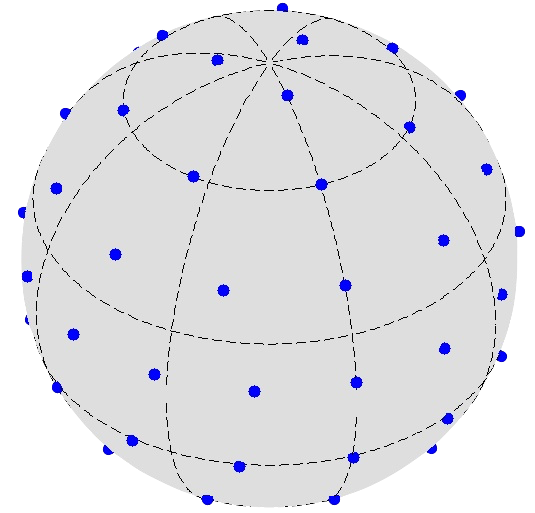# NSHT: Novel optimal sampling spherical harmonic transformsNSHT implements a novel sampling scheme to accurately sample a signal band-limited at $L$ in $L^2$ samples.

Whereas sampling theorems on the sphere require at best $\sim 2 L^2$ samples (see A novel sampling theorem on the sphere; implemented in SSHT), NSHT sampling achieves the optimal sampling rate specified by the dimensionality of band-limited signals in harmonic space. However, a theoretically exact sampling theorem in not obtained.

Nevertheless, NSHT provides functionality to compute fast spherical harmonic transforms for signals sampled according to the optimal sampling scheme developed.